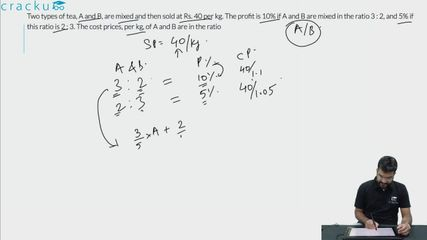Question 76

# Two types of tea, A and B, are mixed and then sold at Rs. 40 per kg. The profit is 10% if A and B are mixed in the ratio 3 : 2, and 5% if this ratio is 2 : 3. The cost prices, per kg, of A and B are in the ratio

Solution

The selling price of the mixture is Rs.40/kg.
Let a be the price of 1 kg of tea A in the mixture and b be the price per kg of tea B.
It has been given that the profit is 10% if the 2 varieties are mixed in the ratio 3:2
Let the cost price of the mixture be x.
It has been given that 1.1x = 40
x = 40/1.1
Price per kg of the mixture in ratio 3:2 = $$\frac{3a+2b}{5}$$
$$\frac{3a+2b}{5} = \frac{40}{1.1}$$
$$3.3a+2.2b=200$$ --------(1)

The profit is 5% if the 2 varieties are mixed in the ratio 2:3.
Price per kg of the mixture in ratio 2:3 = $$\frac{2a+3b}{5}$$
$$\frac{2a+3b}{5} = \frac{40}{1.05}$$
$$2.1a+3.15b=200$$ ------(2)

Equating (1) and (2), we get,
$$3.3a+2.2b = 2.1a+3.15b$$
$$1.2a=0.95b$$
$$\frac{a}{b} = \frac{0.95}{1.2}$$
$$\frac{a}{b} = \frac{19}{24}$$

Therefore, option C is the right answer.

### View Video Solution• All Quant CAT Formulas and shortcuts PDF
• 30+ CAT previous papers with solutions PDF

##### ravitej

1 year, 5 months ago

how come we took sp =1.1*cp. in actual it should be sp = 1.1-cp right. is there any special condition apply here

##### Anurag Majumder

4 years, 2 months ago

Where is 1.1 came from?

##### Here Tuli

3 years, 3 months ago

Cp=sp*100/100+profit this formula has been used here

##### Here Tuli

3 years, 3 months ago

Cp=sp*100/100+profit this formula has been used here